#### Translation

A rigid robot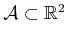is translated by using two parameters,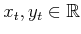. Using definitions from Section 3.2.1,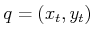, and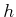is defined as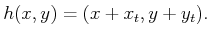(3.25)

A boundary representation of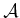can be translated by transforming each vertex in the sequence of polygon vertices using (3.25). Each point,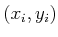, in the sequence is replaced by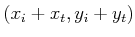.

Now consider a solid representation of, defined in terms of primitives. Each primitive of the form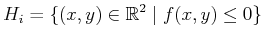(3.26)

is transformed to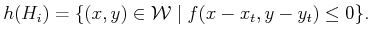(3.27)

Example 3..2 (Translating a Disc)   For example, suppose the robot is a disc of unit radius, centered at the origin. It is modeled by a single primitive,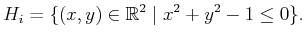(3.28)

Suppose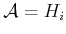is translated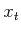units in thedirection and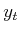units in the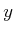direction. The transformed primitive is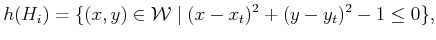(3.29)

which is the familiar equation for a disc centered at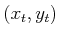. In this example, the inverse,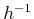is used, as described in Section 3.2.1.The translated robot is denoted as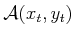. Translation by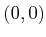is the identity transformation, which results in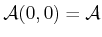, if it is assumed that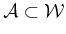(recall thatdoes not necessarily have to be initially embedded in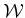). It will be convenient to use the term degrees of freedom to refer to the maximum number of independent parameters that are needed to completely characterize the transformation applied to the robot. If the set of allowable values forandforms a two-dimensional subset of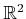, then the degrees of freedom is two.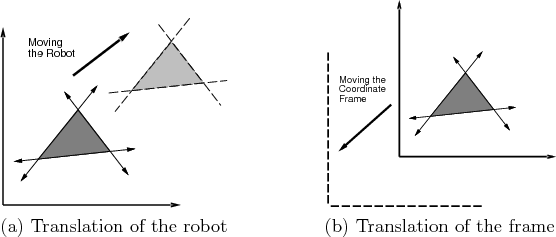Suppose thatis defined directly inwith translation. As shown in Figure 3.7, there are two interpretations of a rigid-body transformation applied to: 1) The world frame remains fixed and the robot is transformed; 2) the robot remains fixed and the world frame is translated. The first one characterizes the effect of the transformation from a fixed world frame, and the second one indicates how the transformation appears from the robot's perspective. Unless stated otherwise, the first interpretation will be used when we refer to motion planning problems because it often models a robot moving in a physical world. Numerous books cover coordinate transformations under the second interpretation. This has been known to cause confusion because the transformations may sometimes appear backward'' from what is desired in motion planning.

Steven M LaValle 2020-08-14